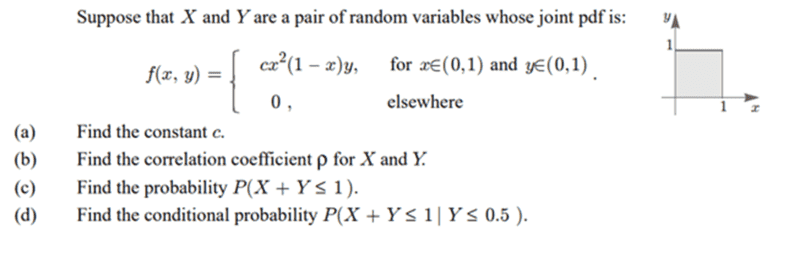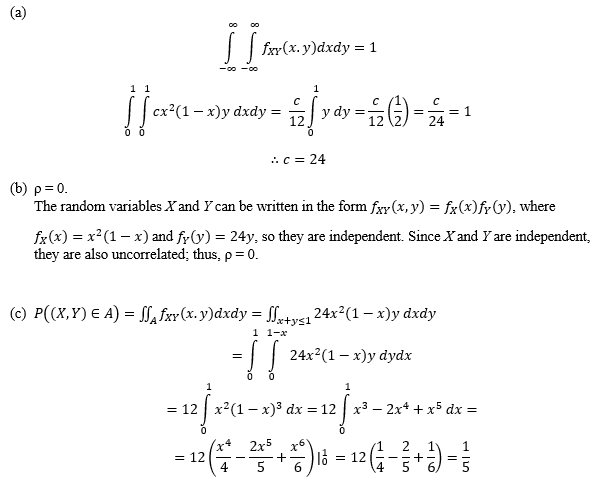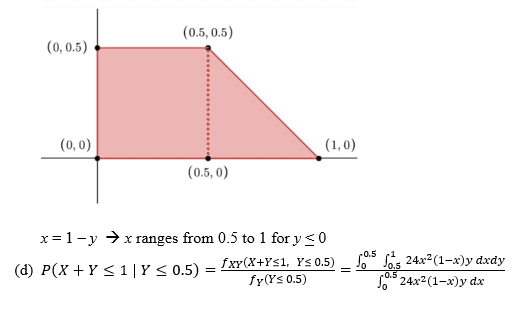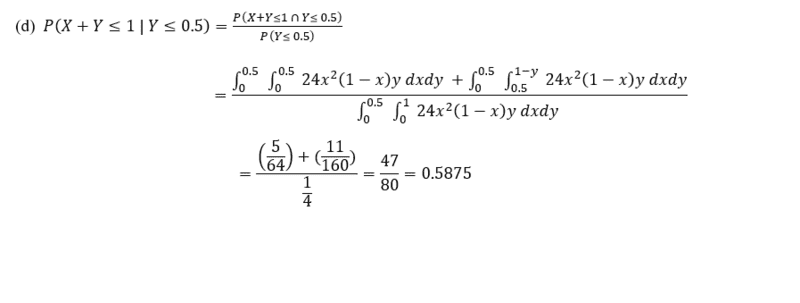# Probability: pair of random variables

• ashah99
In summary, The conversation is about solving a problem involving integration and probability. The group discusses the correct setup for part (d) and provides pointers for the correct implementation. They also confirm the correctness of the answers for parts (a)-(c).f

#### ashah99

Homework Statement
Problem shown below. Various topics related to the joint pdf and RVs
Relevant Equations
Equations used are shown in working attempt below.
Hello all, I would like to check my understanding and get some assistance with last part of the following question, please.
For part (d), would I use f(x | y) = f(x, y) / f(y) ?

Problem statement:My attempt at a solution, not too confident in my set-up for part (d). I drew a sketch of the region of integration.The denominator in the last line must be a double integral, otherwise you can't get a value for it. You need to integrate over the rectangle ##[0,1]\times [0,0.5]##.
You need to break the numerator into two separate integrals: one covering the square on the left and one covering the triangle on the right.

One does not write, as in your central expression on the last line:
$$\frac{f_{XY}(X+Y\leq 1, Y\leq 0.5)} {f_Y(Y\leq 0.5)}$$
You should write something like
$$\frac{Pr(X+Y\leq 1\wedge Y\leq 0.5)} {Pr(Y\leq 0.5)}$$
where the ##Pr## indicates 'probability of' and ##\wedge## means 'and'.

The denominator in the last line must be a double integral, otherwise you can't get a value for it. You need to integrate over the rectangle ##[0,1]\times [0,0.5]##.
You need to break the numerator into two separate integrals: one covering the square on the left and one covering the triangle on the right.

One does not write, as in your central expression on the last line:
$$\frac{f_{XY}(X+Y\leq 1, Y\leq 0.5)} {f_Y(Y\leq 0.5)}$$
You should write something like
$$\frac{Pr(X+Y\leq 1\wedge Y\leq 0.5)} {Pr(Y\leq 0.5)}$$
where the ##Pr## indicates 'probability of' and ##\wedge## means 'and'.

Thank you for the pointers. I very well could still be off on the integral bounds, but if I understood your reply correctly, I get an answer of 0.5875.That's right. Note that you could have written the numerator as
$$\int_0^{1/2}\int_0^{1-y} f(x,y)\,dx\,dy.$$ Your original attempt just had the limits wrong. What @andrewkirk probably had in mind was
$$\int_0^{1/2}\int_0^{1/2} f(x,y)\,dy\,dx + \int_{1/2}^1 \int_0^{1-x} f(x,y)\,dy\,dx$$ The first integral is the square, and the second integral is the triangular region.

That's right. Note that you could have written the numerator as
$$\int_0^{1/2}\int_0^{1-y} f(x,y)\,dx\,dy.$$ Your original attempt just had the limits wrong. What @andrewkirk probably had in mind was
$$\int_0^{1/2}\int_0^{1/2} f(x,y)\,dy\,dx + \int_{1/2}^1 \int_0^{1-x} f(x,y)\,dy\,dx$$ The first integral is the square, and the second integral is the triangular region.

Thank you for your explanation and the integral bounds that you proposed are more intuitive. Do you agree with the final answer for (d)? Also, are my answers for parts (a) - (c) correct? I realize that I have to split the constant c=24 between FX(x) and fY(y) such that both would integrate to 1, i.e. fX (x) = 12x^2(1-x) and fY(y) = 2y.

I got the same answer for (d). The rest looks good as well.